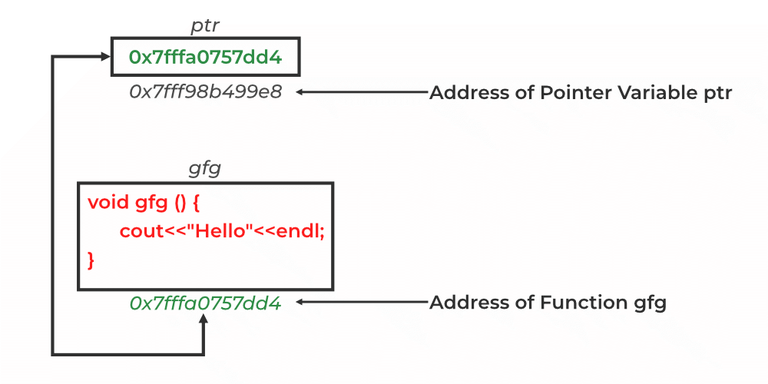GeeksforGeeks App
Open AppBrowser
Continue

Prerequisites:

Pointers are symbolic representations of addresses. They enable programs to simulate call-by-reference as well as to create and manipulate dynamic data structures. Iterating over elements in arrays or other data structures is one of the main use of pointers.

The address of the variable you’re working with is assigned to the pointer variable that points to the same data type (such as an int or string).

Syntax:

`datatype *var_name; `

Address of Function: We all know that every function’s code resides in memory, so every function has an address like all other variables in the program. The name of a function can be used to find the address of the function. We can get the address of a function by just writing the function’s name without parentheses in the function.

### Function Pointer in C++

• The function pointer is used to point functions, similarly, the pointers are used to point variables.
• It is utilized to save a function’s address.
• The function pointer is either used to call the function or it can be sent as an argument to another function.Function Pointer in C++

Syntax

`return_type (*FuncPtr) (parameter type, ....);  `

### Referencing and Dereferencing of the Function Pointer in C++

Similar to the pointer used with variables we perform referencing and dereferencing with a function pointer.

Referencing: When pointer is allocated the address of the function to be associated with it then this process is referred to as referencing.

Dereferencing: When we use the (*)operator  to get the value stored in the pointer.

Syntax:

```// Declaring
return_type (*FuncPtr) (parameter type, ....);

// Referencing
FuncPtr= function_name;

// Dereferencing
data_type x=*FuncPtr;```

## Function pointer used to call the function

In this, we see how we point a pointer to a function and call it using that pointer. It is an efficient way to use

Example:

## C++

 `// C++ program to implementation``// Function Pointer``#include ``using` `namespace` `std;` `int` `multiply(``int` `a, ``int` `b) { ``return` `a * b; }` `int` `main()``{``    ``int` `(*func)(``int``, ``int``);` `    ``// func is pointing to the multiplyTwoValues function` `    ``func = multiply;` `    ``int` `prod = func(15, 2);``    ``cout << ``"The value of the product is: "` `<< prod << endl;` `    ``return` `0;``}`

Output

`The value of the product is: 30`

In the above program, we are declaring a function multiply where we are multiplying two elements a and b, then returning the result. But, rather than directly calling the function we are using a function pointer prod which is doing the same work for us.

## Passing a function pointer as a parameter

When declaring a function pointer to store the memory address of the function but, when we want to pass the return value to the next function. We have two methods to perform this task. First, either pass the value we got or second pass the function pointer that already exists.

Example:

## C++

 `// C++ Program for demonstrating``// function pointer as pointer``#include ``using` `namespace` `std;` `const` `int` `a = 15;``const` `int` `b = 2;` `// Function for Multiplication``int` `multiply() { ``return` `a * b; }` `// Function containing function pointer``// as parameter``void` `print(``int` `(*funcptr)())``{``    ``cout << ``"The value of the product is: "` `<< funcptr()``         ``<< endl;``}` `// Driver Function``int` `main()``{``    ``print(multiply);``    ``return` `0;``}`

Output

`The value of the product is: 30`

Time complexity: O(1).
Auxiliary space: O(1).

In the above program, we are declaring a multiply function in which we multiply 2 variables a and b. We are passing the function pointer as a parameter in the print function, here we use the function pointer to calculate the value from the multiply function and then that value in the print function.

My Personal Notes arrow_drop_up